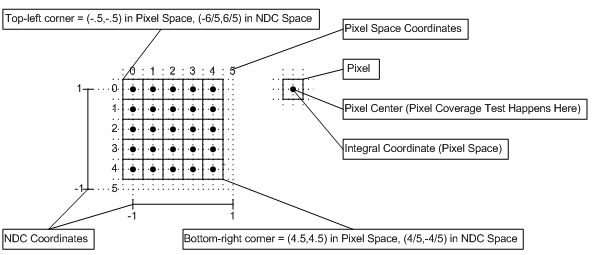# Coordinate Systems (Direct3D 10)

Coordinate systems for Direct3D 10 are defined for pixels and texels.

Differences between Direct3D 9 and Direct3D 10:

• Direct3D 10 defines the upper-left corner of the upper-left pixel as the origin of a render target.
• Direct3D 9 defines the center of the upper-left pixel as the origin of a render target.

Pixel Coordinate System

Texel Coordinate System

Related topics

## Pixel Coordinate System

The pixel coordinate system in Direct3D 10 defines the origin of a render target at the upper-left corner. as shown in the following diagram. Pixel centers are offset by (0.5f,0.5f) from integer locations.### Pixel Coordinate System for Direct3D 9

For reference, here is the pixel coordinate system for Direct3D 9, which defined the origin or a render target as the center of the upper-left pixel, (0.5,0.5) away from the upper left corner, as shown in the following diagram. In Direct3D 9, pixel centers are at integer locations.## Texel Coordinate System

The texel coordinate system has its origin at the top-left corner of the texture, as shown in the following diagram. This makes rendering screen-aligned textures trivial (in Direct3D 10), as the pixel coordinate system is aligned with the texel coordinate system.### Texel Coordinate System

Texture coordinates are represented with either a normalized or a scaled number; each texture coordinate is mapped to a specific texel as follows:

For a normalized coordinate:

• Point sampling: Texel # = floor(U * Width)
• Linear sampling: Left Texel # = floor(U * Width), Right Texel # = Left Texel # + 1

For a scaled coordinate:

• Point sampling: Texel # = floor(U)
• Linear sampling: Left Texel # = floor(U - 0.5), Right Texel # = Left Texel # + 1

Where the width, is the width of the texture (in texels).

Texture address wrapping occurs after the texel location is computed.

Resources (Direct3D 10)